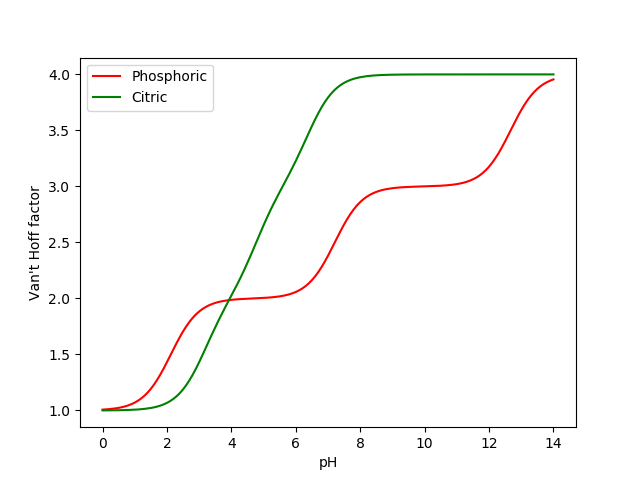Why Does A Potato Battery Work Better Than A Lemon Battery?

posted in: Cool Science, Learn, Videos | 0

Thank you so much for watching this video! If you would like to suggest a video idea please leave it in the comments, and if you enjoyed please like and subscribe! It was my pleasure to add a bit of science to your day!

 The formula for a weak electrolyte: i=1+αi=1+α You can figure out the dissociation factor, αα, from the dissociation constant: K1=[H2A−][H+][H3A]=(αCH3A)2(1−α)CH3A=α21−αCH3AK1=[H2A−][H+][H3A]=(αCH3A)2(1−α)CH3A=α21−αCH3A where CH3ACH3A is the formal concentration of the acid. 2) If you have more species in solution, it will depend on the pH of the solution. In general, you’ll have this expression: i=[H3A]+[H2A−]+[HA2−]+[A3−]+[H+]CH3Ai=[H3A]+[H2A−]+[HA2−]+[A3−]+[H+]CH3A After some tedious substitutions you get i=1+K1[H+]+K1+K2[H+]+K2+K3[H+]+K3i=1+K1[H+]+K1+K2[H+]+K2+K3[H+]+K3 Some relevant values: pH=0→i≈1pH=0→i≈1 pH=pK1→i=1.5pH=pK1→i=1.5 pH=pK2→i=2.5pH=pK2→i=2.5 pH=pK3→i=3.5pH=pK3→i=3.5 pH=14→i≈4pH=14→i≈4 and it changes logarithmically in between.**************
Follow me on instagram: https://www.instagram.com/sciencechase/